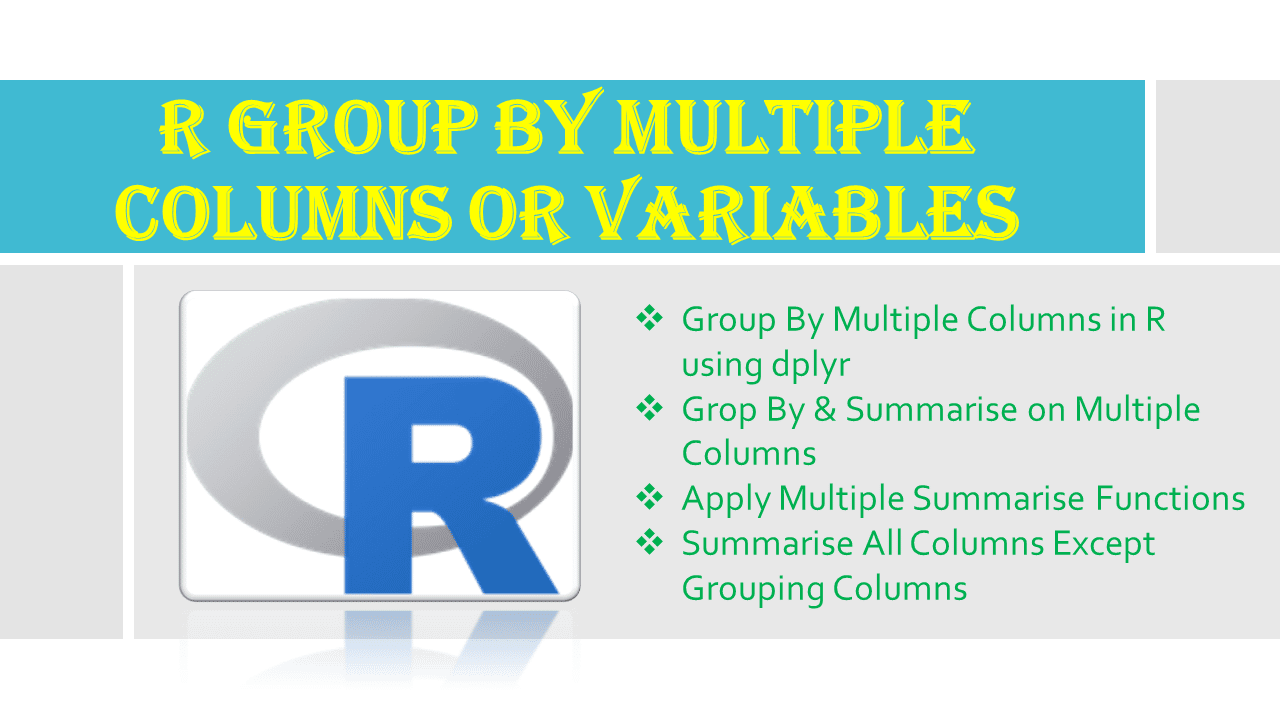# R Group by Multiple Columns or Variables

How to perform a group by on multiple columns in R DataFrame? By using group_by() function from dplyr package we can perform group by on multiple columns or variables (two or more columns) and summarise on multiple columns for aggregations. Later, I will also explain how to apply summarise() on all columns and finally use multiple aggregation functions together.

## 1. Quick Examples of Grouping by Multiple Columns

Following are the quick examples of grouping dataframe on multiple columns.

``````
# Group by on multiple columns
agg_tbl <- df %>% group_by(department, state) %>%
summarise(total_salary=sum(salary))

# Summarise on multiple columns
df2<- df[,c("department","state","salary","bonus")]
agg_tbl <- df2 %>% group_by(department, state) %>%
summarise(across(c(salary, bonus), sum))

# Apply multiple summaries
df2<- df[,c("department","state","salary","bonus")]
agg_tbl <- df2 %>% group_by(department, state) %>%
summarise(across(c(salary, bonus), list(mean = mean, sum = sum)))

# Summarise all columns except grouping columns
df2<- df[,c("department","state","age","salary","bonus")]
agg_tbl <- df2 %>% group_by(department, state) %>%
summarise(across(everything(), list(mean = mean, sum = sum)))
``````
``````
# Read CSV file into DataFrame
df
``````

Yields below output.

## 2. Group By Multiple Columns in R using dplyr

Use group_by() function in R to group the rows in DataFrame by multiple columns (two or more), to use this function, you have to install dplyr first using install.packages(‘dplyr’) and load it using `library(dplyr)`.

All functions in dplyr package take `data.frame` as a first argument. When we use `dplyr` package, we mostly use the infix operator `%>%` from `magrittr`, it passes the left-hand side of the operator to the first argument of the operator’s right-hand side. For example, `x %>% f(y)` converted into `f(x, y)` so the result from the left-hand side is then “piped” into the right-hand side.

I will use infix operator `%>%` across all our examples as the output of group_by() function is input to summarise() function.

``````
library(dplyr)

# Group by on multiple columns
agg_tbl <- df %>% group_by(department, state) %>%
summarise(total_salary=sum(salary))
agg_tbl
``````

Yields below output. This example does the group by on `department` and `state` columns and summarises on `salary` column and applies the `sum` function on each summarised column.

Note that the output of group_by() and summarise() is tibble hence, to convert it to data.frame use `as.data.frame()` function.

``````
df2 <- agg_tbl %>% as.data.frame()
``````

## 3. Grop By & Summarise on Multiple Columns

To perform summarise on multiple columns, create a vector with the column names and use it with across() function.

This example does the group by on `department` and `state` columns, summarises on `salary` & `bonus` columns, and apply the `sum` function on each summarised column.

``````
# Summarise on multiple columns
agg_tbl <- df %>% group_by(department, state) %>%
summarise(across(c(salary, bonus), sum))
``````

Yields below output.

## 4. Apply Multiple Summarise Functions

Similarly, you can also perform multiple aggregation functions on all summarise columns in R.

This example does the group by on `department` and `state` columns, summarises on `salary` and `bonus` columns, and apply the `sum` & `mean` functions on each summarised column.

``````
# Apply multiple summaries
agg_tbl <- df %>% group_by(department, state) %>%
summarise(across(c(salary, bonus), list(mean = mean, sum = sum)))
``````

Yields below output.

## 5. Summarise All Columns Except Grouping Columns

Finally, let’s see how to apply the aggregate functions on all columns of the DataFrame except grouping columns. While doing this make sure your dataframe has only numeric columns plus grouping columns. Having non-numeric on summarise returns an error.

This example does the group by on `department` and `state` columns, summarises on all columns except grouping columns, and apply the `sum` & `mean` functions on all summarised columns.

``````
# Summarise all columns except grouping columns
df2<- df[,c("department","state","age","salary","bonus")]
agg_tbl <- df2 %>% group_by(department, state) %>%
summarise(across(everything(), list(mean = mean, sum = sum)))
agg_tbl
``````

Yields below output.

## 4. Conclusion

In this article, I have explained how to perform group by dataframe on multiple columns and apply different summarising types to get aggregation on grouped data. Since the output of group_by() and summarise() is tibble, use `as.data.frame()` function to convert it to data.frame.

## References

### Naveen (NNK)

Naveen (NNK) is a Data Engineer with 20+ years of experience in transforming data into actionable insights. Over the years, He has honed his expertise in designing, implementing, and maintaining data pipelines with frameworks like Apache Spark, PySpark, Pandas, R, Hive and Machine Learning. Naveen journey in the field of data engineering has been a continuous learning, innovation, and a strong commitment to data integrity. In this blog, he shares his experiences with the data as he come across. Follow Naveen @ @ LinkedIn